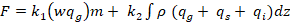# NCMRWF REGIONAL UNIFIED MODEL (NCUM-R)

At the NCMRWF, the regional unified model (NCUM-R) has been operational since 2012 (Mamgain et al. 2017 ) and a current model setup is with a horizontal grid resolution of 4.4km covering Indian domain extended to (-5.98−41°N and 62−106°E) to produce real-time short-range weather forecasts. NCUM-R is described in Jayakumar et al. (2017 & 2018) with the latest tropical science modifications of the first version of Regional Atmosphere (RA1-T) as described in Bush et al. (2017) and is run for 3 days. The vertical coordinate extends up to 38.5 km (80 levels). The regional data assimilation uses most of the conventional and satellite datasets as in the driving global model and additionally assimilates the radial winds only from the radar network (Dutta et al. (2019)).

However, the regional data assimilation is not cyclic and is run only for the single cycle every day from the global model background. It employs the latest version of dynamical core, namely, Even Newer Dynamics for General atmospheric modeling (ENDGame - Wood et al. 2014), run on a rotated latitude-longitude horizontal grid with Arakawa-C staggering and a terrain-following hybrid vertical coordinate with Charney-Philips staggering. It includes a comprehensive set of parameterization schemes including surface/radiation (based on Edwards & Slingo, 1996), blended boundary layer parameterization (Boutle et al. 2014) using a mixing factor of 0.2, large scale cloud parameterization, use a prognostic cloud fraction and condensation (Wilson et al.2008) scheme, cloud microphysics (Wilson and Ballard (1999)) with extensive modification described in Walter et al. 2017. Convection is represented explicitly, i.e. the convection parametrization was completely switched off.

Additional new feature used in this configuration was moisture conservation scheme (Aranami et al., 2015). The model employs 30m resolution Indian Space Research Organisation (ISRO) land use land cover over Indian region along with NASA Shuttle Radar Topographic Mission (SRTM) 90m digital elevation map orography. Land surface and hydrology scheme is JULES land surface scheme (Best et al. 2011; Clark et al. 2011).

For lightning prediction, NCUM-R uses an electric scheme followed by McCaul et al. (2009), which allows in predicting the spatial and temporal distribution of lightning frequency and rate. This electric scheme is not an explicit approach as no electric fields are calculated, nor is it a statistical approach, wherein lightning values are calculated directly from the vertical velocity and hydrometeor masses. It employs two distinct methods to calculate lightning flash-rate density are devised (1) uses the prognostic field vertical air motion multiplied by graupel mixing ratio at the -15oC level (hereafter called as graupel flux), (2) is based on the gridded vertical integral of graupel, snow, and cloud-ice. Therefore, the total model measured lightning flash at each model grid is as follows.where w is vertical air motion, qg is the graupel mixing ratio, and the subscript m attached to the flux implies evaluation at the -15oC level in the mixed-phase region, ρ is the local air density; qg, qs, qi are the simulated mixing ratios of graupel, snow, and cloud-ice, respectively; and k1 and k2 are two empirical constants, k1 = 0.95, and k2 = 0.05. The integration in Eq. (1) is over the full storm depth. Note model calculate the flash rate only when there are storm points. The default threshold to define a “storm” is when model graupel water path (GWP) at grid point exceeds 200 g m-3. In addition this scheme allows to enable/disable the collisions between snow to form graupel.